Forecasting some probability vs. predicting values by some model (e.g., linear regression model).

Goal: Predicting some value (on a scale).

Method: Linear regression.

### 18.3.1 Introduction

This section provides a gentle introduction to linear regression models. Rather than providing a statistical treatment — which typically uses the simple LR model to transition into the realms of multiple predictors, different types of variables and their possible transformations, and non-linear extensions in the GLM framework — we aim to illustrate the principles behind linear regression and key intuitions that are important for any kind of statistical modeling.

Specific goals:

• Understand relation between correlation and simple linear regression models
• Understand common measures to assess model accuracy
• Understand different elements and uses of a model
• Understand the difference between explaining existing (model fitting) data and predicting new data

Historic data by Galton (from first correlation and “regression”):

# install.packages("HistData")
library(HistData)

# ?GaltonFamilies
dim(GaltonFamilies)  # 934 x 8
#>  934   8
# as_tibble(GaltonFamilies)
# View(GaltonFamilies)

GF <- as_tibble(GaltonFamilies)

# Note: The more limited dataset Galton contains
#       abstracted/processed data of 928 parent/child pairs.
# dim(Galton)

Goal: Study the relationship of height between generations, specifically: Association between parent’s and children’s height.

Note: We are tempted to interpret this as causal (i.e., parent’s height as a cause, children’s height as an effect). However, if a relationship exists, we can also view it from the opposite direction: To what extent can parent’s height be predicted by children’s height?

The variable midparentHeight is a weighted average of father and mother, with values of mother multiplied by a factor of 1.08. To account for the fact that men are on average taller than women, Galton “transmuted” (Galton, 1886, p. 247) women into men (see Hanley, 2004, for details). We can verify the calculation of midparentHeight and see the effects of this manipulation by comparing the histograms for all three variables:

# Verify definition of midparentHeight:
mpH <- (GF$father + (1.08 * GF$mother))/2
all.equal(GF$midparentHeight, mpH) #>  TRUE # Compare histograms of 3 distributions: ggplot(GF) + geom_histogram(aes(x = midparentHeight), fill = "grey67", binwidth = 1) + geom_histogram(aes(x = father), fill = "deepskyblue", alpha = .50, binwidth = 1) + geom_histogram(aes(x = mother), fill = "deeppink", alpha = .50, binwidth = 1) + labs(title = "Distribution of height values (for fathers, mothers, vs. midparents)", x = "Height (in inches)", y = "Frequency count", caption = "[Data from HistData::GaltonFamilies]") + theme_ds4psy()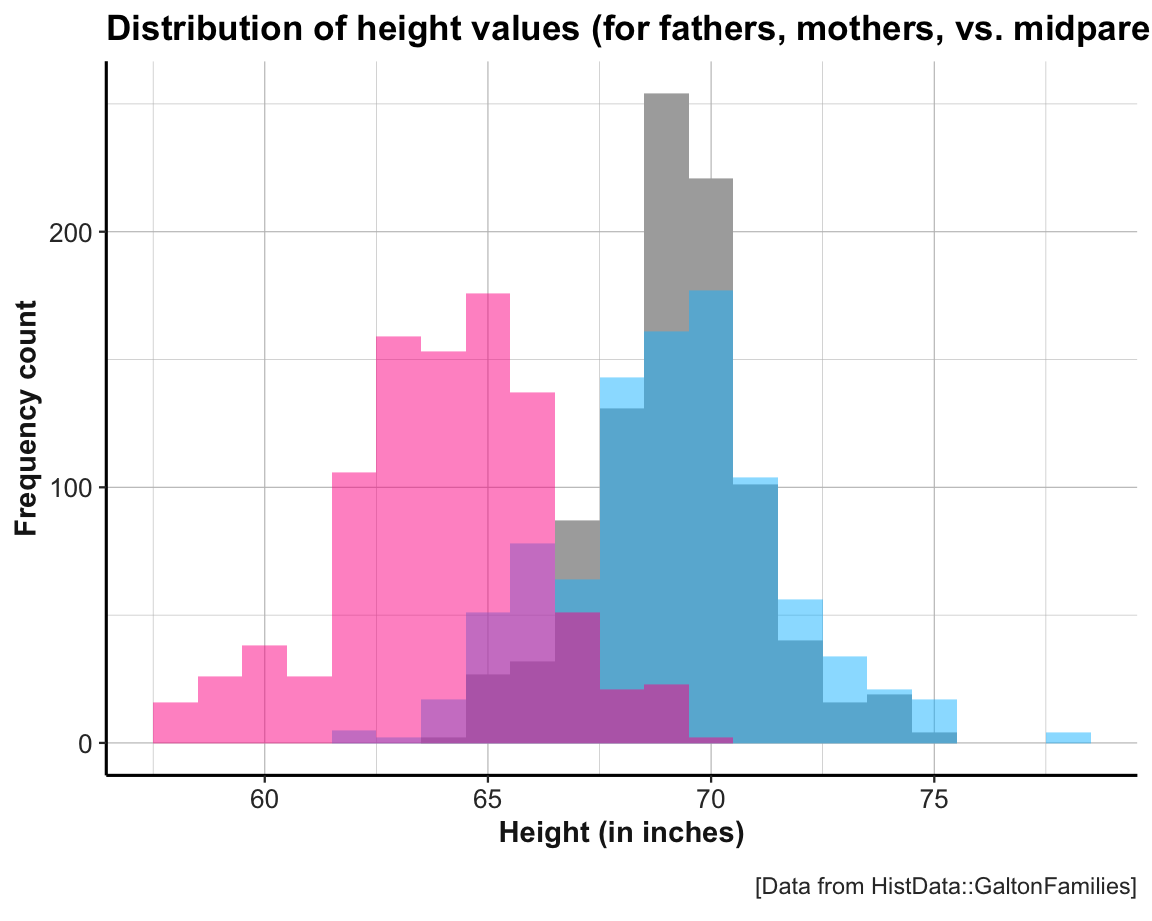Scatterplot of raw values of childHeight as a function of midparentHeight: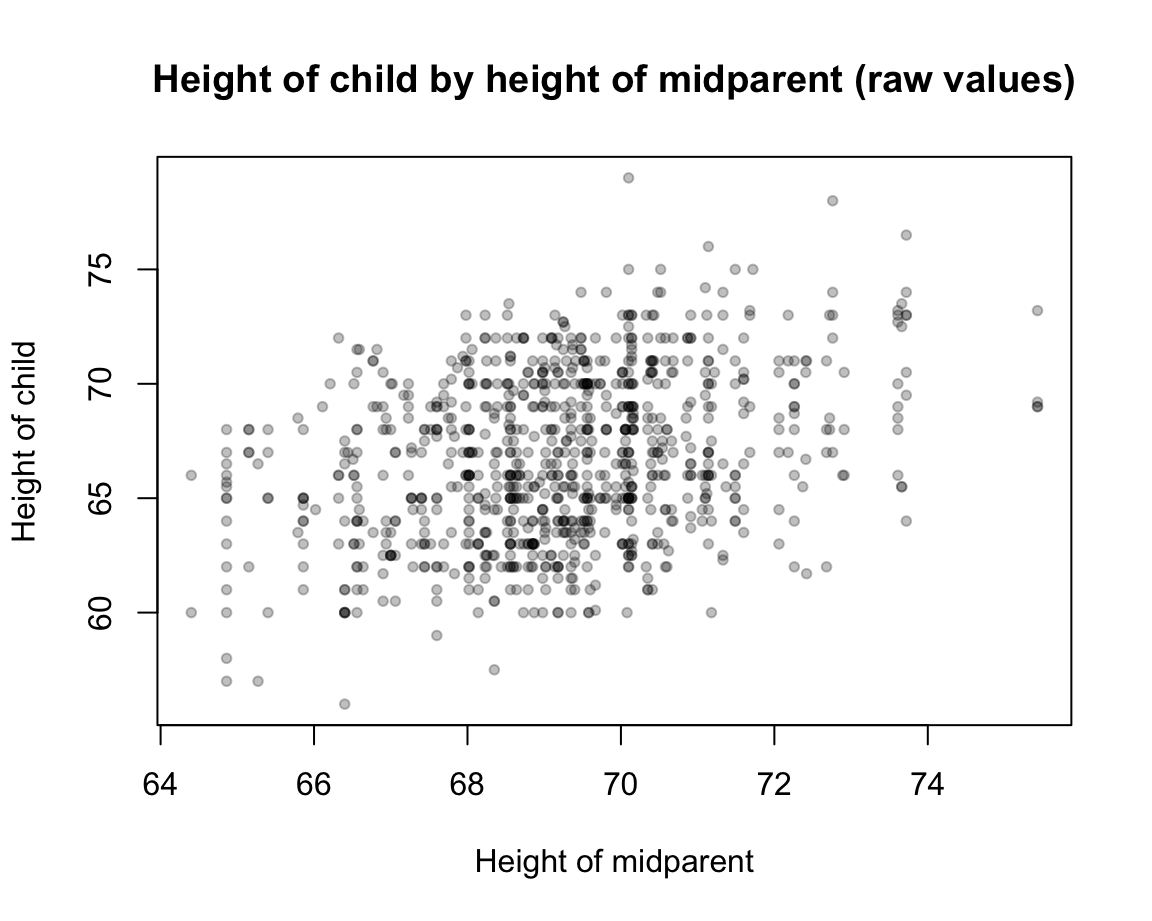Specific task: Explain or predict the values of childHeight by values of midparentHeight. ### 18.3.2 A basic model We want to predict the true values of childHeight $$y_i$$. What would the most simple model be? A null-model for this data: Predict the mean criterion value $$\hat{y_i}$$ for all data points $$x_i$$: For all values of $$x_i$$, predict $$\bar{y_i} = \sum_{i=1}^{n}{y_i}$$. Graphically, this basic model is a horizontal line with a $$y$$-intercept given by the mean criterion value: #>  69.20677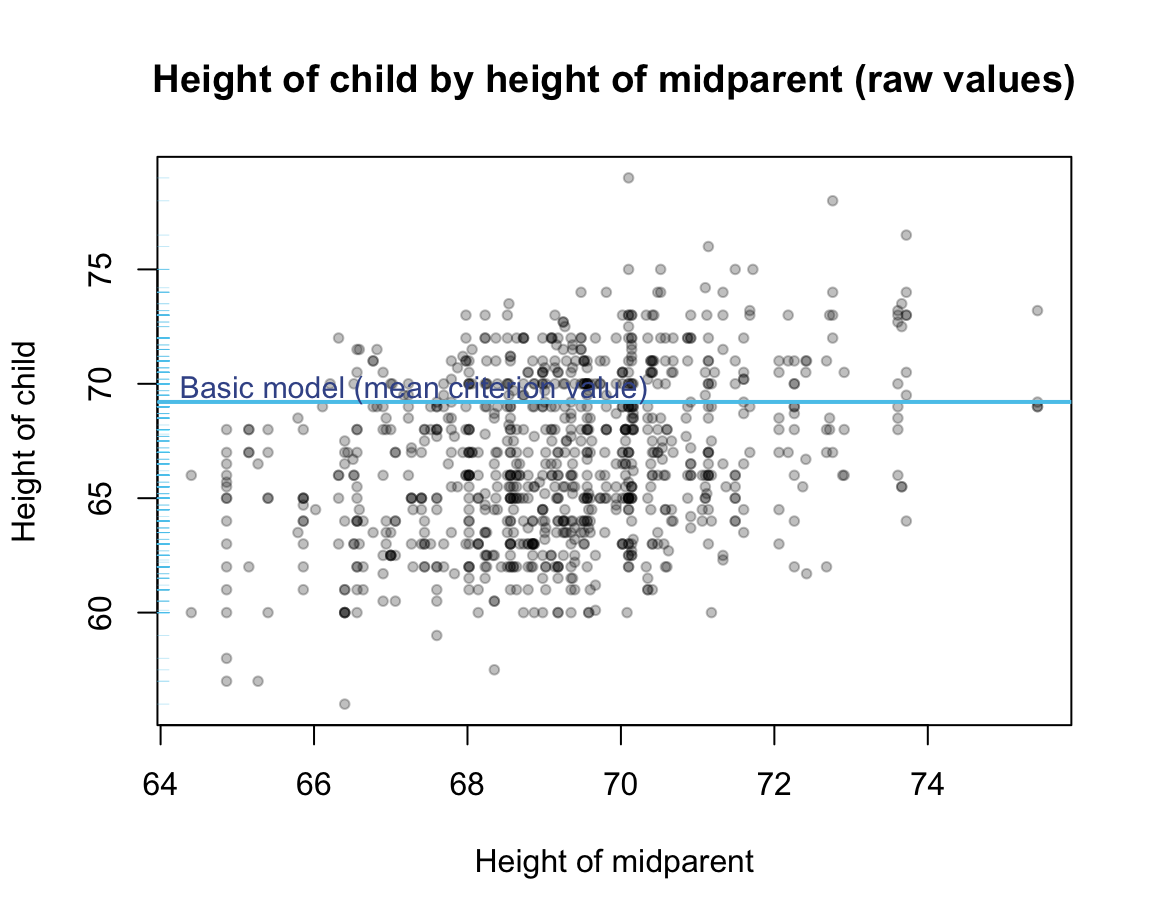Predicting the mean criterion value $$\bar{y_i}$$ for all possible data points $$x_i$$ provides a veritable model. Although this model is primitive and inflexible, it is very simple, as it employs only one parameter (i.e., the $$y$$-intercept of the mean). Nonetheless, it makes a specific prediction for any old or new data point, albeit the rather boring prediction of the same value. How good is this model? We typically evaluate models by the accuracy of their predictions. Given some true criterion values, we can measure model accuracy by quantifying the deviations between these true values $$\hat{y_i}$$ and the corresponding predicted values $$\hat{y_i}$$. Illustration of some deviations between true criterion values and model values (for a random sample of 10 data points):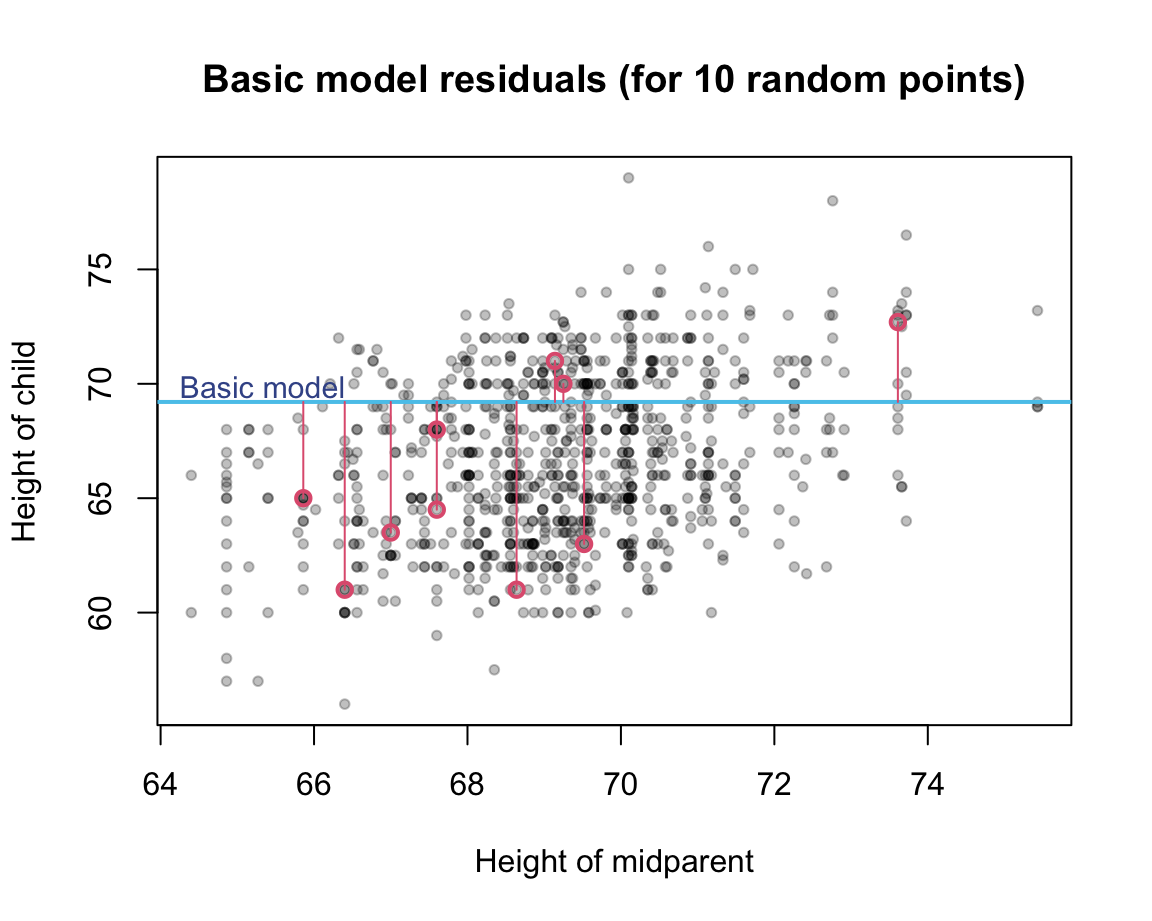As deviations are computed from differences (i.e., true value $$y_i$$ minus predicted value $$\bar{y_i}$$) that can be positive or negative, they are typically squared (so that all differences are positive and larger differences weigh more than smaller ones) and summed (over all data points). Thus, the accuracy of our basic model or the amount of deviation between true data values and the model predictions can be quantified as the total sum of squares (TSS): $\text{TSS} = \sum_{i=1}^{n}{(y_i - \bar{y_i})^2}$ As the value of TSS increases with larger deviations between true and predicted data values, smaller values of TSS indicate better models (i.e., ideally, $$\text{TSS} \rightarrow 0$$). We can express this in R as follows: # Measures of model accuracy: # (see @JamesEtAl2021, p. 69ff.): # Total sum of squares (TSS): ---- TSS <- function(true_vals, pred_vals = NA){ # Special case: if (is.na(pred_vals)){ pred_vals <- mean(true_vals) } sum((true_vals - pred_vals)^2) } and then compute the accuracy of our basic model as: TSS(true_vals = GF$midparentHeight)
#>  3030.886

Thus, the accuracy of our model is measured to be 3030.89. This is definitely not close to our ideal of a deviation value of zero ($$\text{TSS} \rightarrow 0$$). So can we find better models?

As TSS is our first measure of model accuracy, it is important to understand the role of such measures: As the fit between data and model predictions is expressed as a numeric value, we can vary aspects of the model to see whether this improves or decreases the quality of our model (as quantified by this particular measure).

When changing models, we need to distinguish between two aspects:

• varying parameters within a given model type: Changing the value of parameter(s) given by the current model type to optimize the fit of a given model type; vs.

• varying model types: Changing to a different model type (with different numbers or types of parameters).

As our basic model contains only one parameter (the $$y$$-intercept), we could try to predict different values (i.e., different variants of the same model type) and compare the accuracy of these alternative models to the one of our basic model:

TSS(true_vals = GF$midparentHeight, pred_vals = 66) #>  12635.58 TSS(true_vals = GF$midparentHeight, pred_vals = 68)
#>  4391.072
TSS(true_vals = GF$midparentHeight, pred_vals = 70) #>  3618.568 We see that other predictions do not improve further on our basic model (given this measure and model type). Given the nature of our model and our measure, using the mean value as our only model parameter optimizes this particular accuracy measure, i.e., provides the best model when accepting the measure (TSS) and the constraints of our model type (i.e., it being a horizontal line). ### 18.3.3 Linear models How can we improve on this basic model? A more powerful model family: Create linear models with 2 parameters: intercept and slope. $y = a + bx = b_0 + b_1 x$ Generate many random models: Each model generates “predictions” for criterion values. Example: $$y = 0 + 1 x$$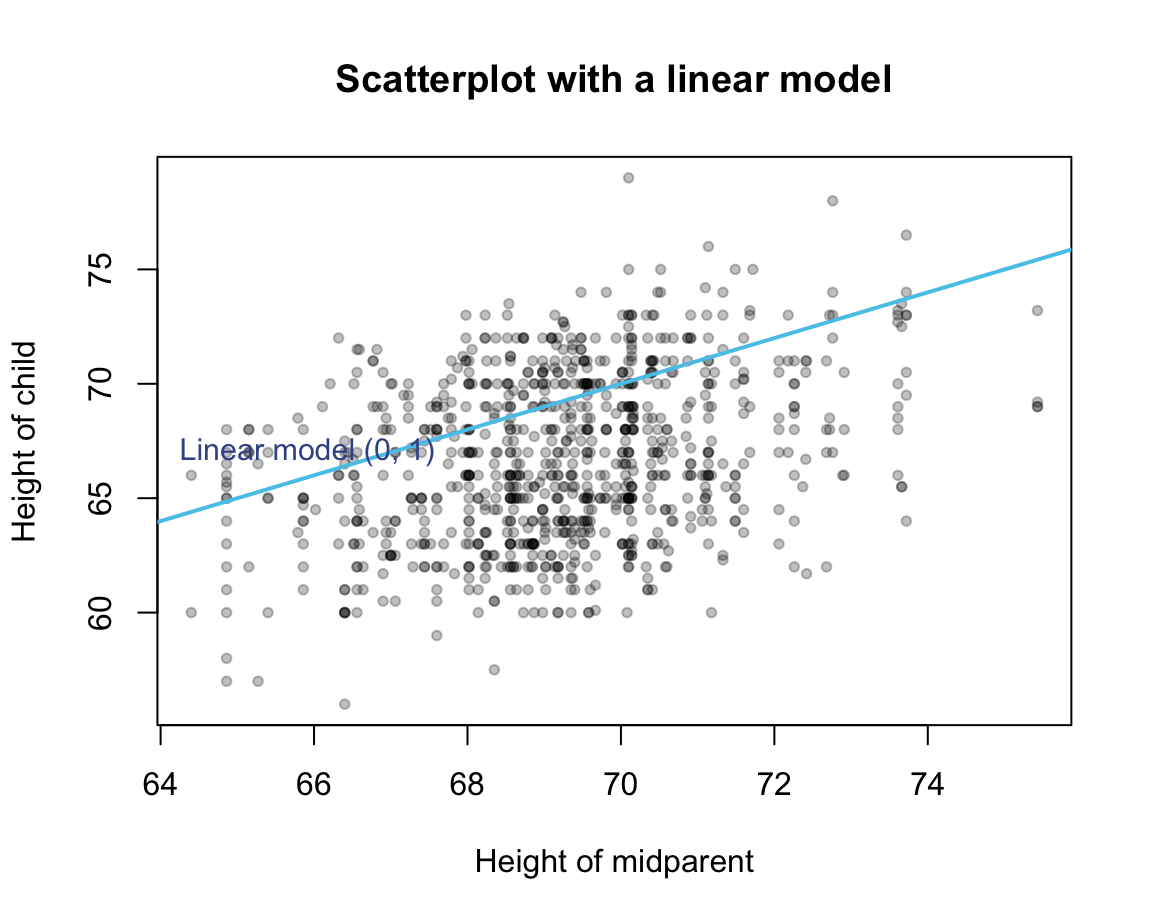Quantify the deviation of the model predictions from the corresponding data point (truth). Goal: Optimize the model by minimizing their accuracy/deviation measure. #### Model accuracy To optimize, we need a measure to quantify model accuracy: For linear models, a basic accuracy measure follows the logic of quantifying the amount of deviation between true and predicted values. This deviation is given by computing the so-called residuals (i.e., the difference between true values $$y_i$$ and predicted values $$\hat{y_i}$$). Illustration of residual values (for a random sample of 10 data points):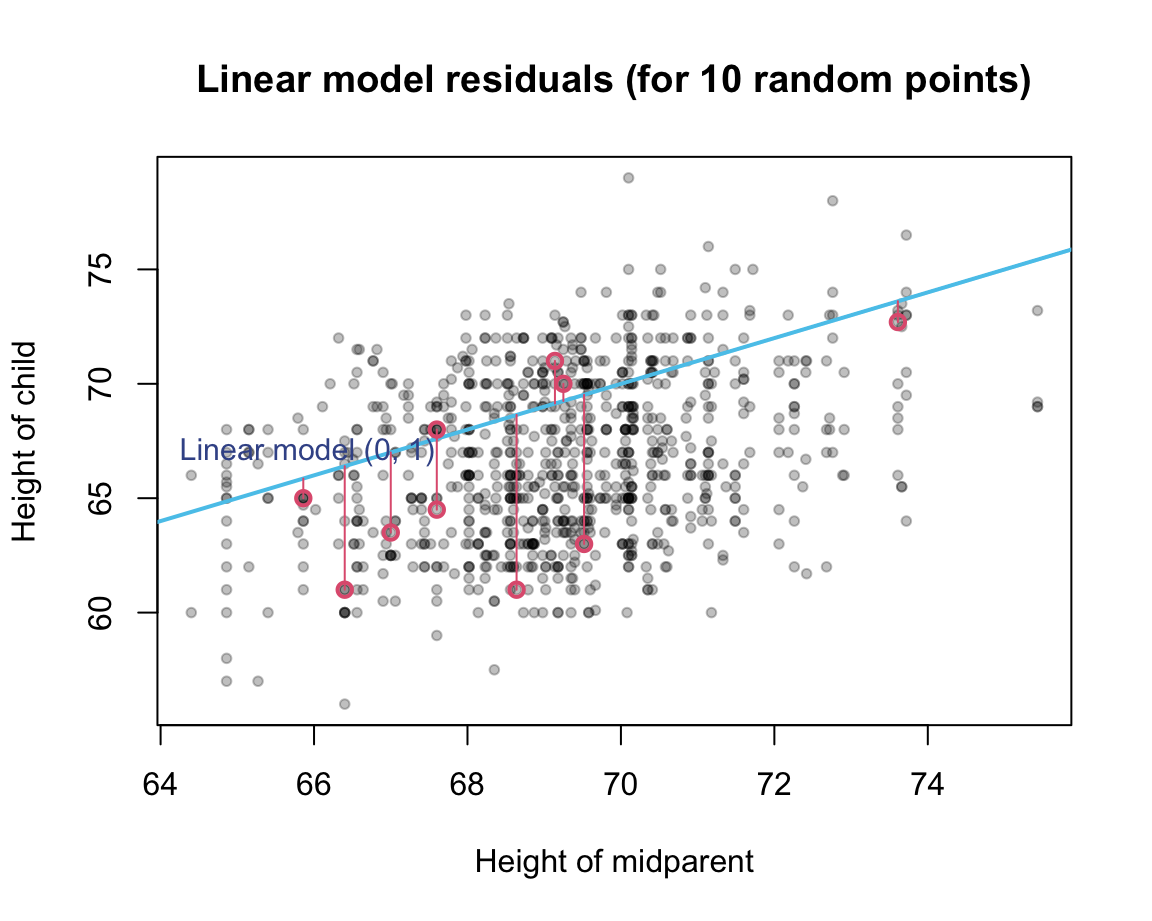Again, residual values exist for every point, can be positive or negative: squaring them, and adding the resulting sums. The resulting accuracy measure is known as the residual sums of squares (RSS, see James et al., 2021, p. 69): $\text{RSS} = \sum_{i=1}^{n}{(y_i - \hat{y_i})^2}$ Note that TSS (above) is but a special case of RSS (with predicting a single value $$\bar{y_i}$$ for all values of $$x_i$$, rather than predicting different values of $$y_i$$ for different values of $$x_i$$) and that RSS will later be used to compute the residual square error (RSE) and combined with TSS to obtain the $$R^2$$-measure. Again, we can easily define an R function for the residual sum of squares (RSS), when providing both the true and predicted values as arguments: # Measures of model accuracy: # (see @JamesEtAl2021, p. 69ff.): # Residual sum of squares (RSS): ---- RSS <- function(true_vals, pred_vals){ sum((true_vals - pred_vals)^2) } Compute RSS for various models… A more systematic approach: Rather than randomly generating parameters and assessing the fit of the corresponding model to the data, we could ask: What would be good values for intercept and slope? Intercept value is arbitrary in the sense that it depends on the scale of the criterion. If the criterion was standardized, the intercept could be zero. Slope represents the linear association between the single predictor and the criterion variable. #### Correlation A measure of linear association: Pearson correlation: (cor_1 <- cor(x = GF$midparentHeight, y = GF$childHeight, method = "pearson")) #>  0.3209499 #### Linear regression Linear regression model: # Linear regression model (raw values): lr_1 <- lm(childHeight ~ midparentHeight, data = GF) summary(lr_1) #> #> Call: #> lm(formula = childHeight ~ midparentHeight, data = GF) #> #> Residuals: #> Min 1Q Median 3Q Max #> -8.9570 -2.6989 -0.2155 2.7961 11.6848 #> #> Coefficients: #> Estimate Std. Error t value Pr(>|t|) #> (Intercept) 22.63624 4.26511 5.307 1.39e-07 *** #> midparentHeight 0.63736 0.06161 10.345 < 2e-16 *** #> --- #> Signif. codes: 0 '***' 0.001 '**' 0.01 '*' 0.05 '.' 0.1 ' ' 1 #> #> Residual standard error: 3.392 on 932 degrees of freedom #> Multiple R-squared: 0.103, Adjusted R-squared: 0.102 #> F-statistic: 107 on 1 and 932 DF, p-value: < 2.2e-16 plot(x = GF$midparentHeight, y = GF$childHeight, pch = 20, col = adjustcolor("black", .25), main = "Height of child by height of midparent (raw values)", xlab = "Height of midparent", ylab = "Height of child") # Plot linear regression line: abline(lr_1, col = "deepskyblue", lwd = 2)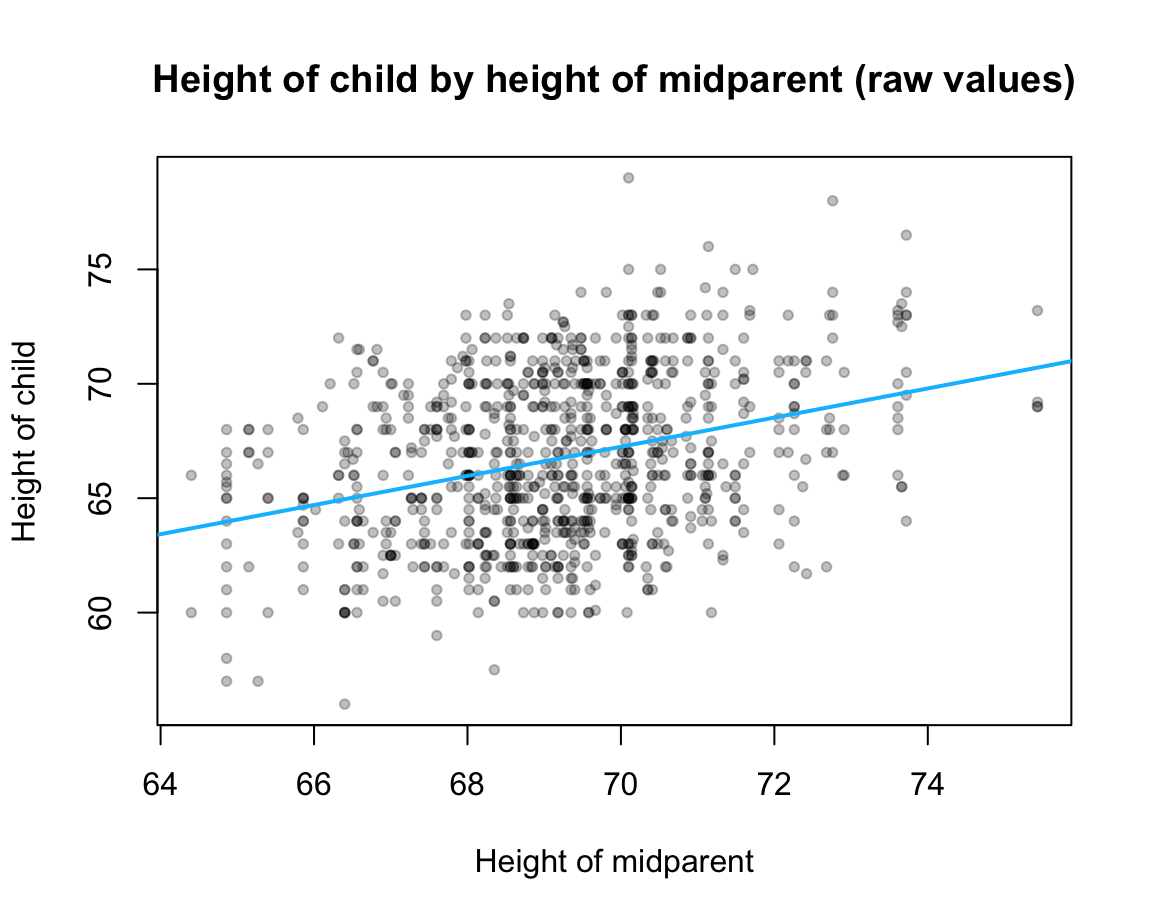Interpretation: Interpreting model coefficients: • Intercept: Can be difficult to interpret, as predictor values of zero often (and here) make no sense. Important to understand that it determines the overall height of the regression line and that it is expressed in units of the predictor variable $$Y$$. • Coefficient of predictor: Indicates the amount of association between this predictor and the criterion, given any other predictors. Value depends on scale and is hard to interpret unless variables were standardized (see below). Importantly, we need to consider value and its SE. If value differs more than 2 SEs from 0, it is significant (unlikely that the observed effect is due to chance alone). As above, we can quantify model accuracy by measuring the deviation of our predicted values (on the linear regression curve) from the true values by RSS: # Compute model predictions (from model coefficients): lr_1_predictions <- coefficients(lr_1) + (coefficients(lr_1) * GF$midparentHeight)

# Note: Model predictions are also provided by lr_1$fitted.values: all.equal(lr_1_predictions, lr_1$fitted.values, check.attributes = FALSE)
#>  TRUE

RSS(true_vals = GF$childHeight, pred_vals = lr_1_predictions) #>  10721.47 Note that RSS value is much lower (i.e., better) than the RSS (or TSS) value of our basic model. (Below, we will use the difference between RSS and TSS to compute a key measure of model accuracy known as the $$R^2$$-statistic.) The values of RSE, $$R^2$$ and $$F$$-statistic in the summary() provide overall measures of model accuracy or quality (and will be defined and explained below). #### Standardizing model variables Standardizing model variables (both criterion and predictor) with the scale() function: # z-Standardization: z_childHeight <- scale(GF$childHeight)

# Histogram:
hist(z_childHeight, col = "grey90", border = "white")

# Show mean value and +/- 1 SD:
abline(v = mean(z_childHeight), col = "deeppink", lwd = 2, lty = 1)
abline(v = mean(z_childHeight) - sd(z_childHeight), col = "deepskyblue", lwd = 1, lty = 2)
abline(v = mean(z_childHeight) + sd(z_childHeight), col = "deepskyblue", lwd = 1, lty = 2)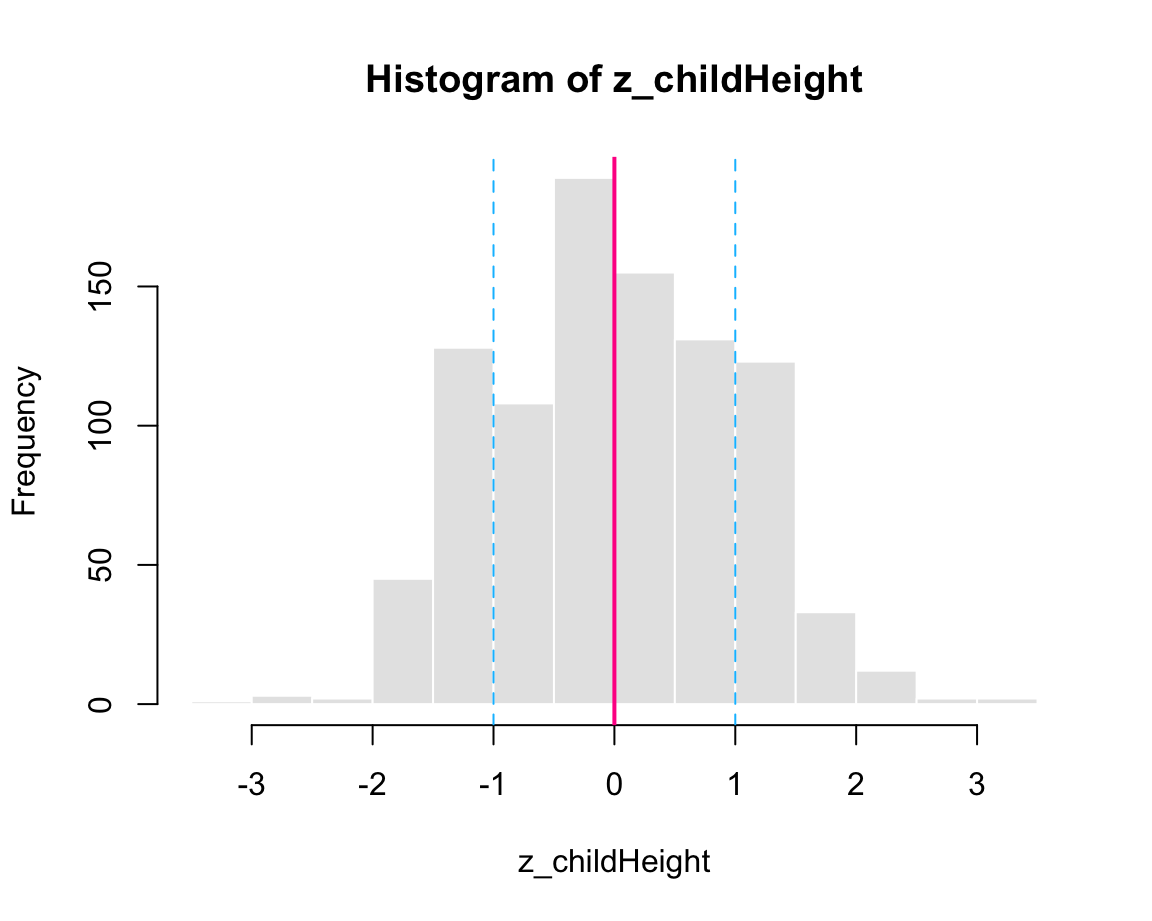z_midparentHeight <- scale(GF$midparentHeight) Standardized model (both criterion and predictor): # Linear regression model (standardized): lr_2 <- lm(scale(childHeight) ~ scale(midparentHeight), data = GF) summary(lr_2) #> #> Call: #> lm(formula = scale(childHeight) ~ scale(midparentHeight), data = GF) #> #> Residuals: #> Min 1Q Median 3Q Max #> -2.5025 -0.7540 -0.0602 0.7812 3.2646 #> #> Coefficients: #> Estimate Std. Error t value Pr(>|t|) #> (Intercept) 7.570e-16 3.101e-02 0.00 1 #> scale(midparentHeight) 3.209e-01 3.102e-02 10.35 <2e-16 *** #> --- #> Signif. codes: 0 '***' 0.001 '**' 0.01 '*' 0.05 '.' 0.1 ' ' 1 #> #> Residual standard error: 0.9476 on 932 degrees of freedom #> Multiple R-squared: 0.103, Adjusted R-squared: 0.102 #> F-statistic: 107 on 1 and 932 DF, p-value: < 2.2e-16 Interpretation: Standardizing the predictor and criterion variables changes their scale and facilitates the interpretation of the coefficients. Importantly, we now need to interpret values in units of standard deviations. In the $$z$$-standardized model lr_2, the intercept value is (near) zero and the regression coefficient of the linear predictor corresponds to the Pearson correlation cor_1 (computed above): near(coefficients(lr_2), 0) #> (Intercept) #> TRUE near(coefficients(lr_2), cor_1) #> scale(midparentHeight) #> TRUE By comparing the summary() outputs for both models, we see that the model’s residual standard error (RSE) changes when rescaling the criterion variable. However, $$R^2$$ and $$F$$-statistic are unchanged by the standardization from lr_1 to lr_2. #### Key elements of a model summary(lr_1) #> #> Call: #> lm(formula = childHeight ~ midparentHeight, data = GF) #> #> Residuals: #> Min 1Q Median 3Q Max #> -8.9570 -2.6989 -0.2155 2.7961 11.6848 #> #> Coefficients: #> Estimate Std. Error t value Pr(>|t|) #> (Intercept) 22.63624 4.26511 5.307 1.39e-07 *** #> midparentHeight 0.63736 0.06161 10.345 < 2e-16 *** #> --- #> Signif. codes: 0 '***' 0.001 '**' 0.01 '*' 0.05 '.' 0.1 ' ' 1 #> #> Residual standard error: 3.392 on 932 degrees of freedom #> Multiple R-squared: 0.103, Adjusted R-squared: 0.102 #> F-statistic: 107 on 1 and 932 DF, p-value: < 2.2e-16 # Note: # str(lr_1) # shows list structure with 12 elements # Important elements: ------ coefficients(lr_1) #> (Intercept) midparentHeight #> 22.6362405 0.6373609 lr_1$coefficients
#>     (Intercept) midparentHeight
#>      22.6362405       0.6373609

# Different types of values: -----

# in data:
GF$midparentHeight # predictor values #>  75.43 75.43 75.43 75.43 73.66 73.66 73.66 73.66 72.06 72.06 72.06 72.06 #>  72.06 72.06 72.06 69.09 69.09 69.09 69.09 69.09 69.09 73.72 73.72 73.72 #>  73.72 73.72 73.72 73.72 72.91 72.91 72.91 72.89 72.37 70.48 70.48 70.48 #>  70.48 70.48 70.48 70.48 70.48 69.94 72.68 72.68 72.68 72.68 72.41 72.41 #>  72.41 71.60 71.60 71.60 71.60 71.60 71.60 71.60 71.60 71.60 71.33 71.33 #>  71.33 71.33 71.33 71.33 71.06 71.06 71.06 70.62 73.61 73.61 73.61 73.61 #>  73.61 73.61 73.61 #> [ reached getOption("max.print") -- omitted 859 entries ] GF$childHeight      # criterion values
#>   73.2 69.2 69.0 69.0 73.5 72.5 65.5 65.5 71.0 68.0 70.5 68.5 67.0 64.5 63.0
#>  72.0 69.0 68.0 66.5 62.5 62.5 69.5 76.5 74.0 73.0 73.0 70.5 64.0 70.5 68.0
#>  66.0 66.0 65.5 74.0 70.0 68.0 67.0 67.0 66.0 63.5 63.0 65.0 71.0 62.0 68.0
#>  67.0 71.0 70.5 66.7 72.0 70.5 70.2 70.2 69.2 68.7 66.5 64.5 63.5 74.0 73.0
#>  71.5 62.5 66.5 62.3 66.0 64.5 64.0 62.7 73.2 73.0 72.7 70.0 69.0 68.5 68.0
#>  [ reached getOption("max.print") -- omitted 859 entries ]

# in model:
lr_1$fitted.values #> 1 2 3 4 5 6 7 8 #> 70.71237 70.71237 70.71237 70.71237 69.58424 69.58424 69.58424 69.58424 #> 9 10 11 12 13 14 15 16 #> 68.56447 68.56447 68.56447 68.56447 68.56447 68.56447 68.56447 66.67150 #> 17 18 19 20 21 22 23 24 #> 66.67150 66.67150 66.67150 66.67150 66.67150 69.62249 69.62249 69.62249 #> 25 26 27 28 29 30 31 32 #> 69.62249 69.62249 69.62249 69.62249 69.10622 69.10622 69.10622 69.09348 #> 33 34 35 36 37 38 39 40 #> 68.76205 67.55744 67.55744 67.55744 67.55744 67.55744 67.55744 67.55744 #> 41 42 43 44 45 46 47 48 #> 67.55744 67.21326 68.95963 68.95963 68.95963 68.95963 68.78754 68.78754 #> 49 50 51 52 53 54 55 56 #> 68.78754 68.27128 68.27128 68.27128 68.27128 68.27128 68.27128 68.27128 #> 57 58 59 60 61 62 63 64 #> 68.27128 68.27128 68.09919 68.09919 68.09919 68.09919 68.09919 68.09919 #> 65 66 67 68 69 70 71 72 #> 67.92711 67.92711 67.92711 67.64667 69.55238 69.55238 69.55238 69.55238 #> 73 74 75 #> 69.55238 69.55238 69.55238 #> [ reached getOption("max.print") -- omitted 859 entries ] lr_1$residuals
#>           1           2           3           4           5           6
#>  2.48762699 -1.51237301 -1.71237301 -1.71237301  3.91575578  2.91575578
#>           7           8           9          10          11          12
#> -4.08424422 -4.08424422  2.43553321 -0.56446679  1.93553321 -0.06446679
#>          13          14          15          16          17          18
#> -1.56446679 -4.06446679 -5.56446679  5.32849508  2.32849508  1.32849508
#>          19          20          21          22          23          24
#> -0.17150492 -4.17150492 -4.17150492 -0.12248587  6.87751413  4.37751413
#>          25          26          27          28          29          30
#>  3.37751413  3.37751413  0.87751413 -5.62248587  1.39377645 -1.10622355
#>          31          32          33          34          35          36
#> -3.10622355 -3.09347633 -3.26204866  6.44256343  2.44256343  0.44256343
#>          37          38          39          40          41          42
#> -0.55743657 -0.55743657 -1.55743657 -4.05743657 -4.55743657 -2.21326168
#>          43          44          45          46          47          48
#>  2.04036946 -6.95963054 -0.95963054 -1.95963054  2.21245690  1.71245690
#>          49          50          51          52          53          54
#> -2.08754310  3.72871923  2.22871923  1.92871923  1.92871923  0.92871923
#>          55          56          57          58          59          60
#>  0.42871923 -1.77128077 -3.77128077 -4.77128077  5.90080667  4.90080667
#>          61          62          63          64          65          66
#>  3.40080667 -5.59919333 -1.59919333 -5.79919333 -1.92710589 -3.42710589
#>          67          68          69          70          71          72
#> -3.92710589 -4.94666709  3.64762382  3.44762382  3.14762382  0.44762382
#>          73          74          75
#> -0.55237618 -1.05237618 -1.55237618
#>  [ reached getOption("max.print") -- omitted 859 entries ]

# Note:
# (1) The fitted.values are equal to the predicted criterion values, given
#     the raw values of the predictor and the model (coefficients):
model_predictions <- lr_1$coefficients + lr_1$coefficients * GF$midparentHeight all.equal(model_predictions, lr_1$fitted.values, check.attributes = FALSE)
#>  TRUE

# (2) The residuals are equal to raw values (of criterion) minus fitted.values:
model_errors <- GF$childHeight - lr_1$fitted.values
true_pred_vals  <- lr_3$fitted.values # Model accuracy: RSE(true_train_vals, true_pred_vals) #>  3.481212 Rsq(true_train_vals, true_pred_vals) #>  0.09122942 # summary(lr_3) +++ here now +++ Next: Checking prediction accuracy for unseen TEST data GF_test Distinguish: explaining old data vs. predicting new data ToDo: Next steps: • Elements of a model (e.g., str()) • Fitting vs. predicting (explaining old vs. predicting new data) • Regression to the mean (Galton’s discovery/point) • Comparing models (ANOVA) • Multiple predictors (collinearity?) ### 18.3.4 Comparing models Using the GaltonFamilies (GF) data, we can try alternative predictors: Rather than using the complex variable midparentHeight as our predictor, we can compare and contrast regressions using either father or mother as a single predictor (using standardized models): # Using data from GF: # Above, we computed: # (0) Linear regression model on midparentHeight (standardized): summary(lr_2) #> #> Call: #> lm(formula = scale(childHeight) ~ scale(midparentHeight), data = GF) #> #> Residuals: #> Min 1Q Median 3Q Max #> -2.5025 -0.7540 -0.0602 0.7812 3.2646 #> #> Coefficients: #> Estimate Std. Error t value Pr(>|t|) #> (Intercept) 7.570e-16 3.101e-02 0.00 1 #> scale(midparentHeight) 3.209e-01 3.102e-02 10.35 <2e-16 *** #> --- #> Signif. codes: 0 '***' 0.001 '**' 0.01 '*' 0.05 '.' 0.1 ' ' 1 #> #> Residual standard error: 0.9476 on 932 degrees of freedom #> Multiple R-squared: 0.103, Adjusted R-squared: 0.102 #> F-statistic: 107 on 1 and 932 DF, p-value: < 2.2e-16 # Consider 2 alternatives: # (1) Linear regression model on father height (standardized): lr_2_f <- lm(scale(childHeight) ~ scale(father), data = GF) summary(lr_2_f) #> #> Call: #> lm(formula = scale(childHeight) ~ scale(father), data = GF) #> #> Residuals: #> Min 1Q Median 3Q Max #> -2.8737 -0.7648 -0.0636 0.7477 3.3374 #> #> Coefficients: #> Estimate Std. Error t value Pr(>|t|) #> (Intercept) -3.581e-16 3.156e-02 0.000 1 #> scale(father) 2.660e-01 3.158e-02 8.425 <2e-16 *** #> --- #> Signif. codes: 0 '***' 0.001 '**' 0.01 '*' 0.05 '.' 0.1 ' ' 1 #> #> Residual standard error: 0.9645 on 932 degrees of freedom #> Multiple R-squared: 0.07078, Adjusted R-squared: 0.06978 #> F-statistic: 70.99 on 1 and 932 DF, p-value: < 2.2e-16 # (2) Linear regression model on mother height (standardized): lr_2_m <- lm(scale(childHeight) ~ scale(mother), data = GF) summary(lr_2_m) #> #> Call: #> lm(formula = scale(childHeight) ~ scale(mother), data = GF) #> #> Residuals: #> Min 1Q Median 3Q Max #> -2.6632 -0.7326 -0.0405 0.8001 3.3436 #> #> Coefficients: #> Estimate Std. Error t value Pr(>|t|) #> (Intercept) 4.653e-16 3.207e-02 0.000 1 #> scale(mother) 2.013e-01 3.209e-02 6.275 5.36e-10 *** #> --- #> Signif. codes: 0 '***' 0.001 '**' 0.01 '*' 0.05 '.' 0.1 ' ' 1 #> #> Residual standard error: 0.9801 on 932 degrees of freedom #> Multiple R-squared: 0.04053, Adjusted R-squared: 0.0395 #> F-statistic: 39.37 on 1 and 932 DF, p-value: 5.362e-10 Both alternatives show significant correlations between predictor and criterion, but appear to be worse than the combined model lr_2. This raises the question: • Are the models different from each other? Comparing models: First, ensure that models of interest are based on the same cases (i.e., no missing or NA values on individual variables): sum(is.na(GF$midparentHeight))
#>  0
sum(is.na(GF$father)) #>  0 sum(is.na(GF$mother))
#>  0

No missing values here, so we can compare model fits. (Otherwise, we would need to filter the data to only contain cases with values on all predictors.)

Comparing models essentially asks: Is one model better than another? To answer this, we first need to ask: Better in which respect? Explanatory power in terms of accuracy vs. other factors (complexity)?

Here: Complexity is the same, hence we can only compare these models in terms of accuracy. Two possible approaches:

1. Amount of variance explained: Does one model explain more variance than another?
# Comparing models: Rsq
summary(lr_2)$r.squared #>  0.1030088 summary(lr_2_f)$r.squared
#>  0.0707765
summary(lr_2_m)$r.squared #>  0.04053053 1. $$F$$-test between models: Differences in accuracy between models (based on the same data) can be checked by conducting an $$F$$-test between models, using the anova() function: # Comparing models: F-test between models anova(lr_2, lr_2_f) #> Analysis of Variance Table #> #> Model 1: scale(childHeight) ~ scale(midparentHeight) #> Model 2: scale(childHeight) ~ scale(father) #> Res.Df RSS Df Sum of Sq F Pr(>F) #> 1 932 836.89 #> 2 932 866.97 0 -30.073 anova(lr_2, lr_2_m) #> Analysis of Variance Table #> #> Model 1: scale(childHeight) ~ scale(midparentHeight) #> Model 2: scale(childHeight) ~ scale(mother) #> Res.Df RSS Df Sum of Sq F Pr(>F) #> 1 932 836.89 #> 2 932 895.19 0 -58.292 anova(lr_2_f, lr_2_m) #> Analysis of Variance Table #> #> Model 1: scale(childHeight) ~ scale(father) #> Model 2: scale(childHeight) ~ scale(mother) #> Res.Df RSS Df Sum of Sq F Pr(>F) #> 1 932 866.97 #> 2 932 895.19 0 -28.22 Interpretation: The 3 models do not differ significantly from each other, but lr_2 explains a higher proportion of variance than the others. Can we boost the performance by using more complex models (e.g., including multiple predictors)? Next: Creating linear models with more than one predictor… ### 18.3.5 Multiple predictors Using both father and mother as individual predictors: # Using data from GF: # Above, we computed: # (0) Linear regression model on midparentHeight (standardized): summary(lr_2) #> #> Call: #> lm(formula = scale(childHeight) ~ scale(midparentHeight), data = GF) #> #> Residuals: #> Min 1Q Median 3Q Max #> -2.5025 -0.7540 -0.0602 0.7812 3.2646 #> #> Coefficients: #> Estimate Std. Error t value Pr(>|t|) #> (Intercept) 7.570e-16 3.101e-02 0.00 1 #> scale(midparentHeight) 3.209e-01 3.102e-02 10.35 <2e-16 *** #> --- #> Signif. codes: 0 '***' 0.001 '**' 0.01 '*' 0.05 '.' 0.1 ' ' 1 #> #> Residual standard error: 0.9476 on 932 degrees of freedom #> Multiple R-squared: 0.103, Adjusted R-squared: 0.102 #> F-statistic: 107 on 1 and 932 DF, p-value: < 2.2e-16 # Consider alternative model with 2 predictors: # (1) Linear regression model on father height (standardized): lr_2_fm <- lm(scale(childHeight) ~ scale(father) + scale(mother), data = GF) summary(lr_2_fm) #> #> Call: #> lm(formula = scale(childHeight) ~ scale(father) + scale(mother), #> data = GF) #> #> Residuals: #> Min 1Q Median 3Q Max #> -2.5472 -0.7658 -0.0609 0.7728 3.2671 #> #> Coefficients: #> Estimate Std. Error t value Pr(>|t|) #> (Intercept) 1.059e-16 3.098e-02 0.000 1 #> scale(father) 2.548e-01 3.106e-02 8.204 7.66e-16 *** #> scale(mother) 1.859e-01 3.106e-02 5.987 3.05e-09 *** #> --- #> Signif. codes: 0 '***' 0.001 '**' 0.01 '*' 0.05 '.' 0.1 ' ' 1 #> #> Residual standard error: 0.9469 on 931 degrees of freedom #> Multiple R-squared: 0.1052, Adjusted R-squared: 0.1033 #> F-statistic: 54.74 on 2 and 931 DF, p-value: < 2.2e-16 Overall model fit (as measured by RSE and $$R^2$$) almost identical — which is not surprising, given that the midparentheight predictor of lr_2 is a linear combination of mother and father. Deciding between models is tricky: Conceptually, they make different points (e.g., lr_2_fm quantifies the partial relationships between childrenHeight to both the father and mother predictors, while lr_2 glosses over the differences between parents). At the same time, lr_2_fm is more complex without gaining anything in terms of accuracy. Comparing model fit while also considering their complexity: Using information critera (e.g., Akaikes Information Criterion, AIC): AIC(lr_2, lr_2_fm) #> df AIC #> lr_2 3 2554.042 #> lr_2_fm 4 2553.733 Here, hardly any difference. Generally, models with lower AIC are preferred. Hence, both conceptual clarity and AIC suggest that lr_2_fm may be better here (although our models essentially make the same predictions). Using multiple predictors raises an important new question: • Are predictors highly correlated? Here: Are the heights of father and mother highly correlated? This would be the case if height was a systematic criterion in human mate search. Important assumption of LR with multiple predictors: Avoiding collinearity between predictors (so-called multicollinearity). 1. Correlation between predictor variables: cor(GF$father, GF$mother, method = "pearson") #>  0.06036612 Value close to zero: No substantial correlation. (Incidentally, this point was addressed (and negated) by Galton as well.) 1. Checking multicollinearity of a model: The variance inflation factor (VIF) for each variable should have a value close to 1, and not above 10: library(car) car::vif(lr_2_fm) #> scale(father) scale(mother) #> 1.003657 1.003657 Here: No indication of multicollinearity or variance inflation. #### 18.3.5.1 Practice Here are some tasks to check your understanding of predicting continuous variables using linear regression models: 1. Scatterplot and linear model: Create the scatterplot and the corresponding linear regression line for the GaltonFamilies data (from HistData) and the standardized linear regression model lr_2 (for explaining the criterion childHeight by one predictor midparentHeight). Solution: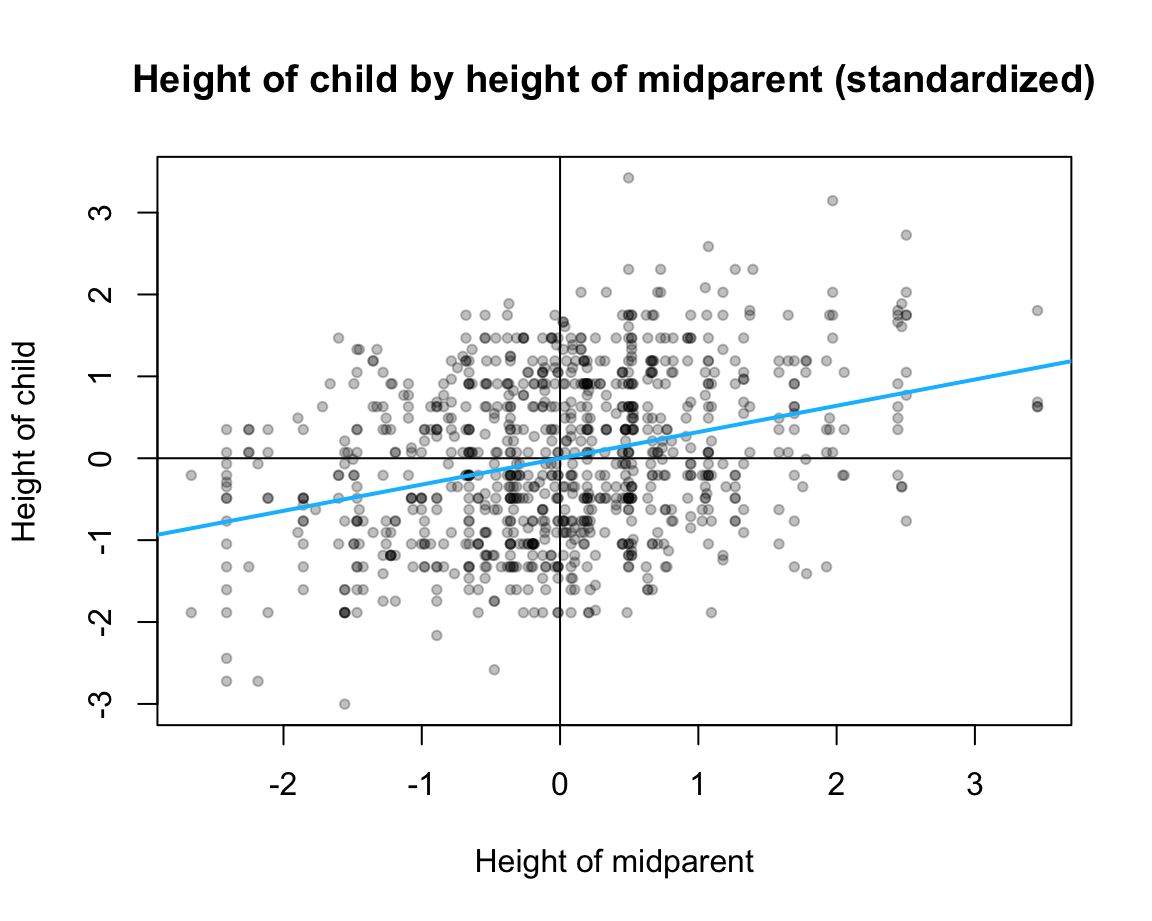1. Cross-validation: Reverse the roles of GF_train and GF_test by first fitting a linear model to the GF_test data and then predicting new values for the GF_train data. ### 18.3.6 Older parts The following notes are based on Chapter 23: Model basics of the r4ds book . This chapter — and the following ones on 24: Model building and 25: Many models — provide a decent introduction to general modeling principles (e.g., starting with scatterplot, model evaluation by distance function, random vs. optimized models, different optimization methods, different types of linear models, e.g., with categorical predictors and interactions). However, the statistical principles are somewhat obscured by using tidyverse syntax and the modelr package. Hence, we recommend translating the important concepts of the chapter into base R. #### Introduction The two parts of modeling: 1. Defining a family of models that express a precise, but generic, pattern that you want to capture (e.g., as an equation that contains free parameters). For instance, linear models (that predict y from x) have the general form y = p1 + p2 * x and two parameters: an intercept p1 and a slope p2. 2. Generating a fitted model by finding the model from the family that is the closest to our data (assuming some distance measure). Thus, the “best” model is not “true,” but the “closest” based on some criterion of distance. Code from Chapter 23: Model basics of the r4ds book : # setup: library(tidyverse) library(modelr) library(ds4psy) library(unikn) options(na.action = na.warn) # data: dat <- modelr::sim1 dim(dat) # 30 x 2 #>  30 2 Figure 18.1 shows a scatterplot of data: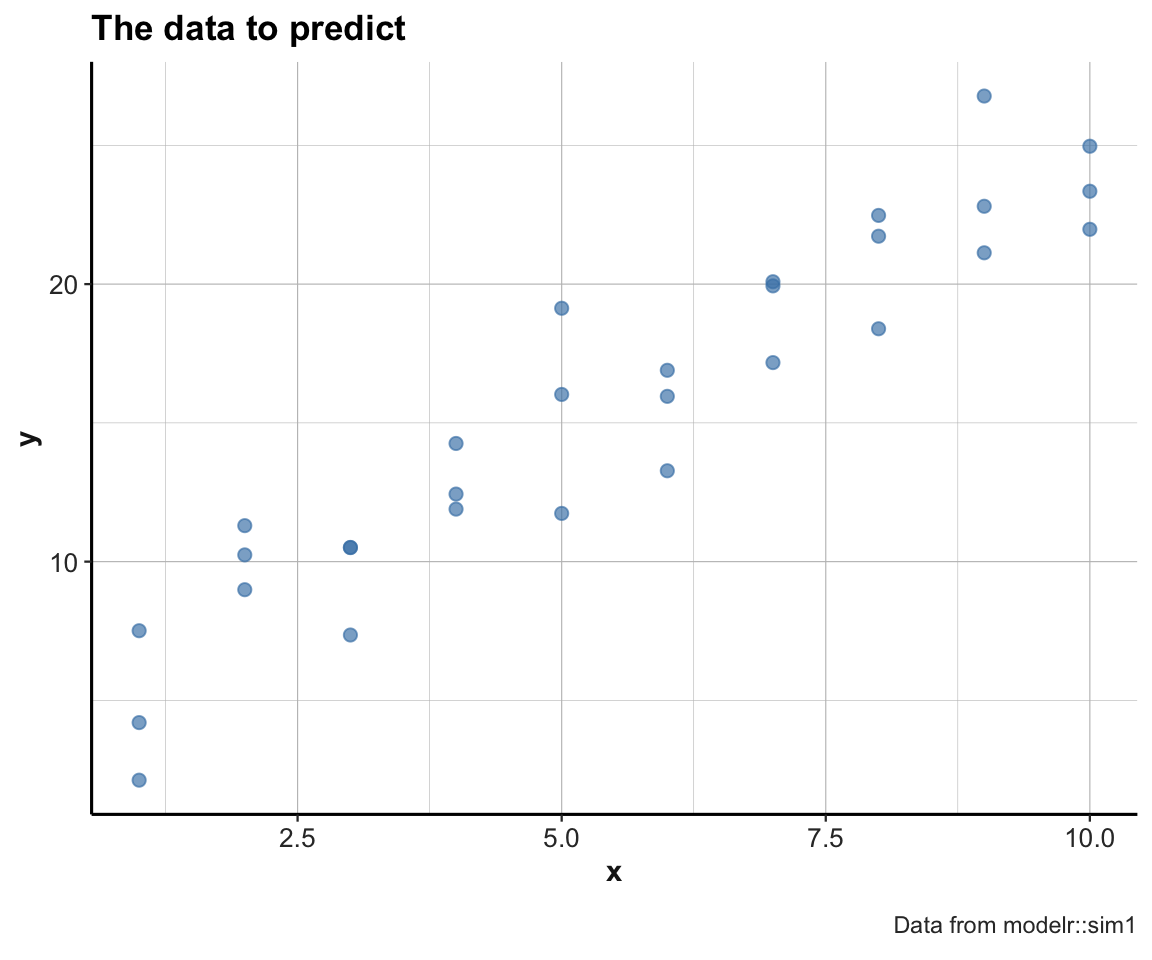Figure 18.1: A scatterplot of the to-be-predicted data. #### Random models Generate n linear models of form y = p1 + p2 * x: n <- 100 set.seed(246) # for reproducible randomness. # Sample n random values for p1 and p2: models <- tibble( p1 = runif(n, -20, 20), p2 = runif(n, -5, 5) ) ggplot(sim1, aes(x, y)) + geom_abline(aes(intercept = p1, slope = p2), data = models, alpha = 1/5) + geom_point(color = "steelblue", size = 2, alpha = 2/3) + labs(title = "The data to predict and random linear models", caption = "Data from modelr::sim1") + theme_ds4psy()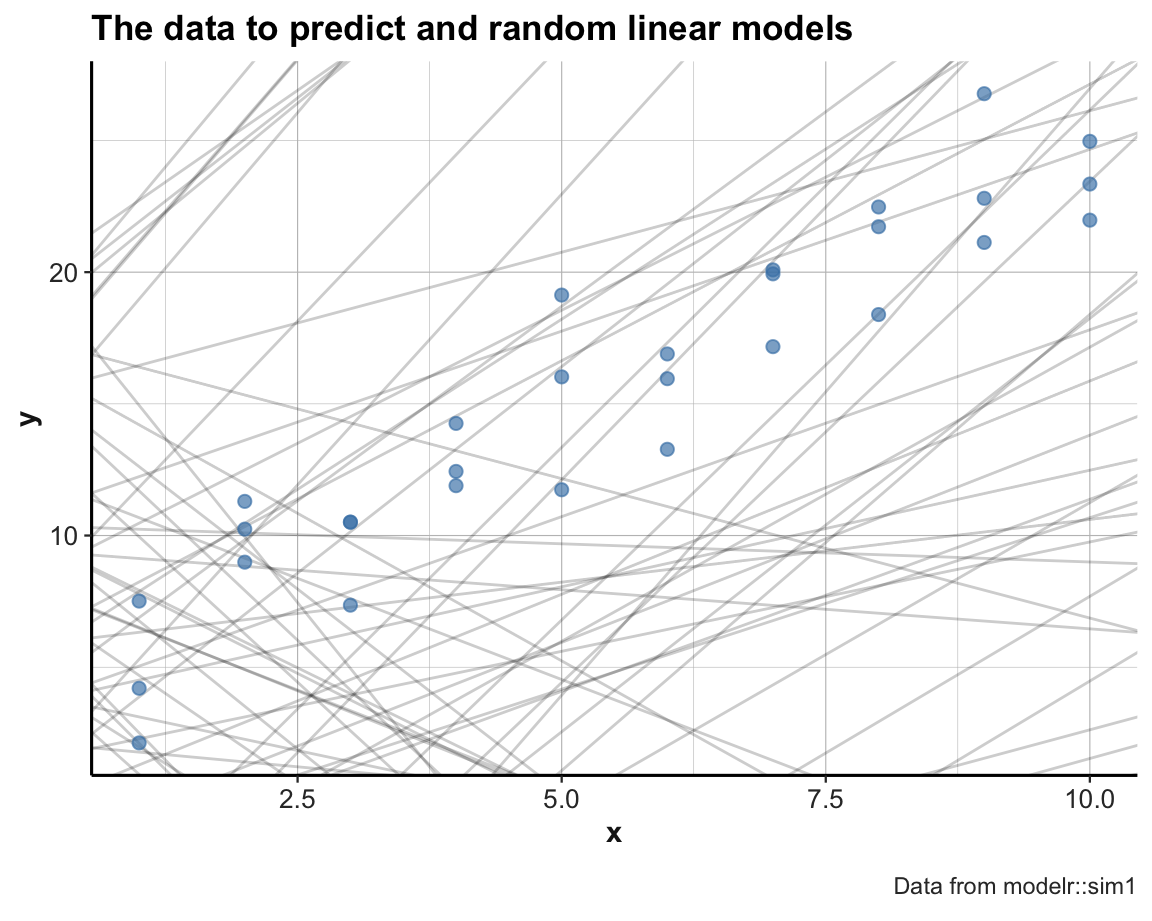Note usage of set.seed() for reproducible randomness. Some random linear models appear to be ok, but most of them are pretty bad (i.e., do not describe the locations and relations of the data points very well). We can improve the quality of our models by narrowing down the range of parameters from which we sample: set.seed(123) # for reproducible randomness. # Sample n random values for p1 and p2: models <- tibble( p1 = runif(n, 0, 10), p2 = runif(n, 0, 5) ) ggplot(sim1, aes(x, y)) + geom_abline(aes(intercept = p1, slope = p2), data = models, alpha = 1/5) + geom_point(color = "steelblue", size = 2, alpha = 2/3) + labs(title = "The data to predict and random linear models", caption = "Data from modelr::sim1") + theme_ds4psy()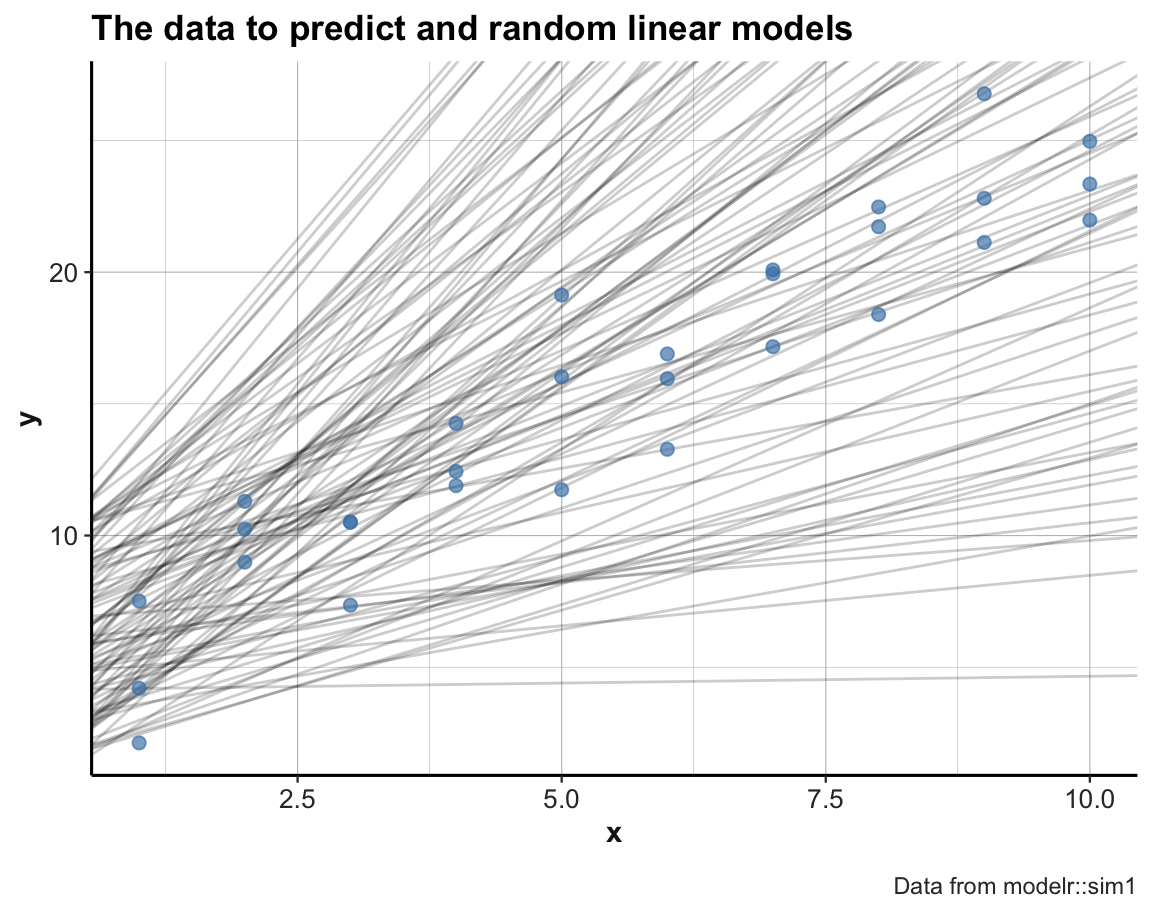The new set of models is much better. But to determine which is the best candidate among them, we need some way of comapring them to the data in a quantitative fashion. To measure the performance of a model, we need to quantify the distance between the model and the actual data points. A function that computes the predictions of a model (i.e., the model’s y values for some input data data$x), given the model parameters mp:

# mp ... model parameters

# Model predictions:
model1 <- function(model, data) {
model + model * data$x } # Generate predictions for various model parameters and dat: model1(c(6, 3), dat) #>  9 9 9 12 12 12 15 15 15 18 18 18 21 21 21 24 24 24 27 27 27 30 30 30 33 #>  33 33 36 36 36 model1(c(6, 2), dat) #>  8 8 8 10 10 10 12 12 12 14 14 14 16 16 16 18 18 18 20 20 20 22 22 22 24 #>  24 24 26 26 26 model1(c(5, 3), dat) #>  8 8 8 11 11 11 14 14 14 17 17 17 20 20 20 23 23 23 26 26 26 29 29 29 32 #>  32 32 35 35 35 model1(c(5, 2), dat) #>  7 7 7 9 9 9 11 11 11 13 13 13 15 15 15 17 17 17 19 19 19 21 21 21 23 #>  23 23 25 25 25 Adding a distance function: root of the mean-squared deviation: The following function computes the difference between actual and predicted, square them, average them, and the take the square root: distance <- function(model, data) { diff <- data$y - model1(model, data)
sqrt(mean(diff ^ 2))
}

# Measure distance for models and dat:
distance(c(6, 3), dat)
#>  7.803283
distance(c(6, 2), dat)
#>  2.60544
distance(c(5, 3), dat)
#>  6.920964
distance(c(5, 2), dat)
#>  2.190166

Alternative distance function (using 2 model parameters as two distinct arguments and only using the data of dat):

dist <- function(p1, p2) {
distance(c(p1, p2), data = dat)
}

# Checks:
all.equal(dist(6, 3), distance(c(6, 3), dat))
#>  TRUE
all.equal(dist(6, 2), distance(c(6, 2), dat))
#>  TRUE

We can now compute the distance of each model in models:

# using a for loop:
md <- rep(NA, nrow(models))

for (i in 1:nrow(models)){
md[i] <- dist(models$p1[i], models$p2[i])
}
md

# Or directly:
md_2 <- purrr::map2_dbl(models$p1, models$p2, dist)

# Verify equality:
all.equal(md, md_2)

In one step: Compute the distance of all models (generated above) and arrange by lowest distance:

models <- models %>%
mutate(dist = purrr::map2_dbl(p1, p2, dist)) %>%
arrange(dist)

models  # with a column for dist
#> # A tibble: 100 × 3
#>      p1    p2  dist
#>   <dbl> <dbl> <dbl>
#> 1  4.15  2.05  2.13
#> 2  1.88  2.33  2.41
#> 3  2.32  2.20  2.43
#> 4  6.53  1.61  2.48
#> 5  5.51  2.05  2.50
#> 6  6.93  1.60  2.50
#> # … with 94 more rows

We can now select the top_n models (i.e., those with the lowest overall distance to the sim1 data) and compare them to the data:

# Plot the top models:
top_n <- 10

# subset of models to plot:
m2p <- models[1:top_n, ]  # base R solution (given that models are sorted by -dist)
m2p <- dplyr::filter(models, rank(dist) <= top_n)  # dplyr solution
# m2p

pt <- paste0("The data to predict and the top ", top_n, " random linear models")

ggplot(sim1, aes(x, y)) +
geom_abline(aes(intercept = p1, slope = p2, color = dist),
data = m2p, alpha = 4/5) +
geom_point(color = "steelblue", size = 2, alpha = 2/3) +
labs(title = pt, caption = "Data from modelr::sim1") +
scale_color_gradient(low = "gold", high = Bordeaux) +
theme_ds4psy()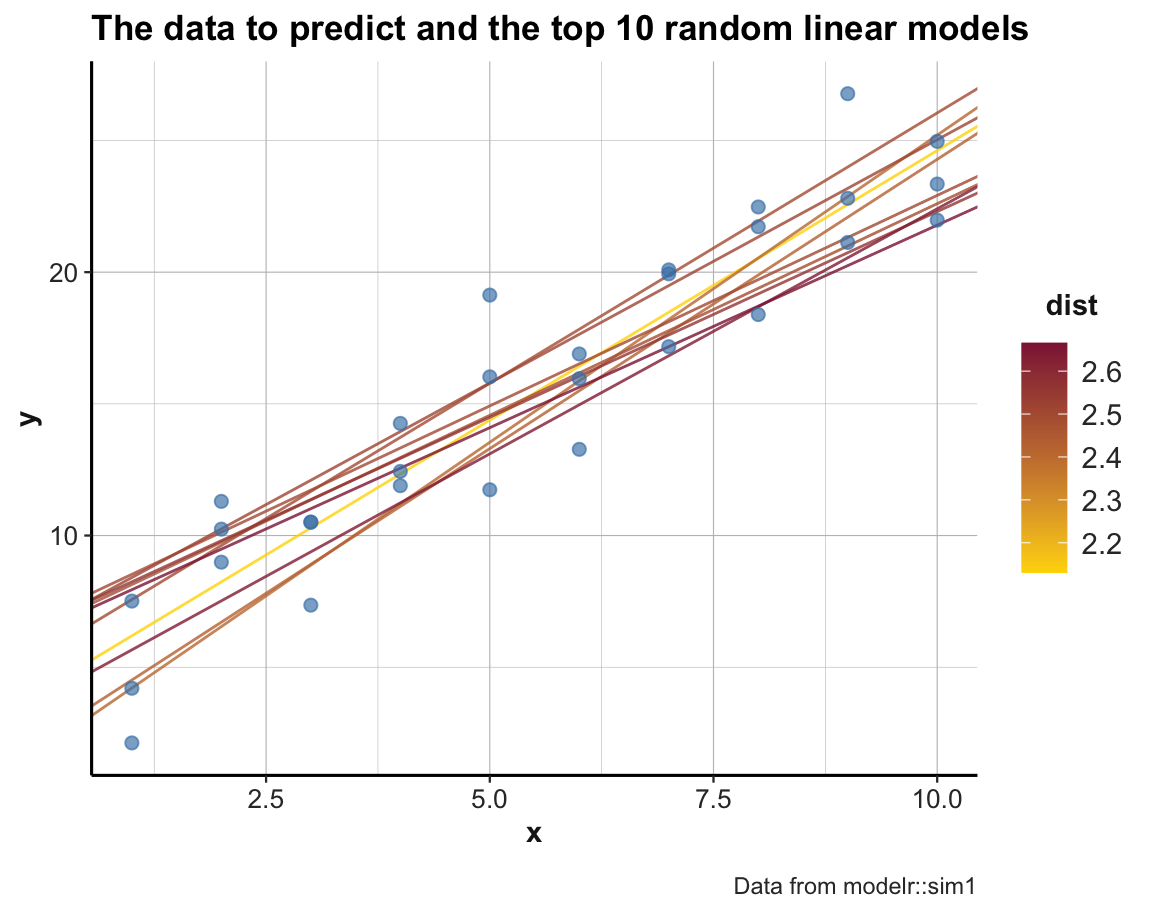An alternative way of looking at the models is by plotting the parameter pair p1 and p2 of each model as a scatterplot. To gain an impression about a model’s performance, we can color the corresponding point by -dist (i.e., models with a lower distance value will be colored in a lighter color). Additionally, the following command first draws the subset of the top_n models in a larger size and brighter color, effectively highlighting those models in the full scatterplot:

ggplot(models, aes(p1, p2)) +
geom_point(data = filter(models, rank(dist) <= top_n),
size = 4, color = Seegruen) +
geom_point(aes(color = dist), size = 2) +
scale_color_gradient(low = "gold", high = Bordeaux) +
theme_ds4psy()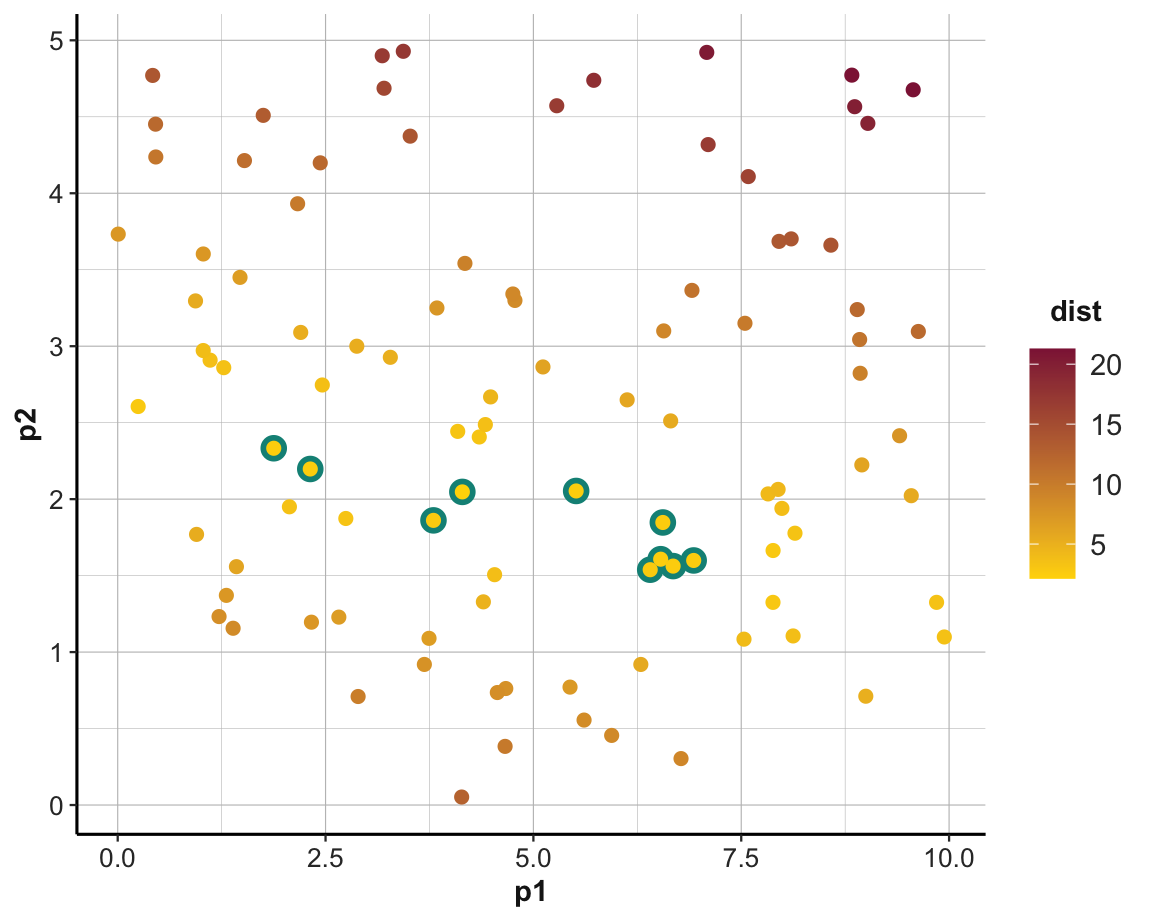#### Minimizing distance

An important part of quantitative modeling is to find parameters that would optimize (i.e., maximize or minimize) some measure.

There are multiple ways of doing this.

If we are considering a one-dimensional range of values, the optimize() function (of the stats package) searches this range (from some lower to some upper value) for a minimum (or maximum) of the first argument of some function f:

curve(expr = (x - 1/3)^2, from = -1, to = 2, col = "deepskyblue", lwd = 2, ylab = "y")

# As a function:
fn <- function(x, a) {(x - a)^2}  # x as 1st parameter (to be optimized)

(mn <- optimize(fn, c(-1, 2), a = 1/3))
#> $minimum #>  0.3333333 #> #>$objective
#>  0
(mx <- optimize(fn, c(-1, 2), a = 1/3, maximum = TRUE))
#> $maximum #>  1.999924 #> #>$objective
#>  2.777525
(mx_2 <- optimize(fn, c(-1, 1), a = 1/3, maximum = TRUE)) # Note limited range
#> $maximum #>  -0.999959 #> #>$objective
#>  1.777668

# Not as lists:
# (mx <- unlist(mx))  # as named vector

points(x = mn$minimum, y = mn$objective, col = "olivedrab3", pch = 16, cex = 2)     # using mn
points(x = mx$maximum, y = mx$objective, col = "brown2", pch = 17, cex = 2)         # using mx
points(x = mx_2$maximum, y = mx_2$objective, col = "steelblue", pch = 18, cex = 2)  # using mx_2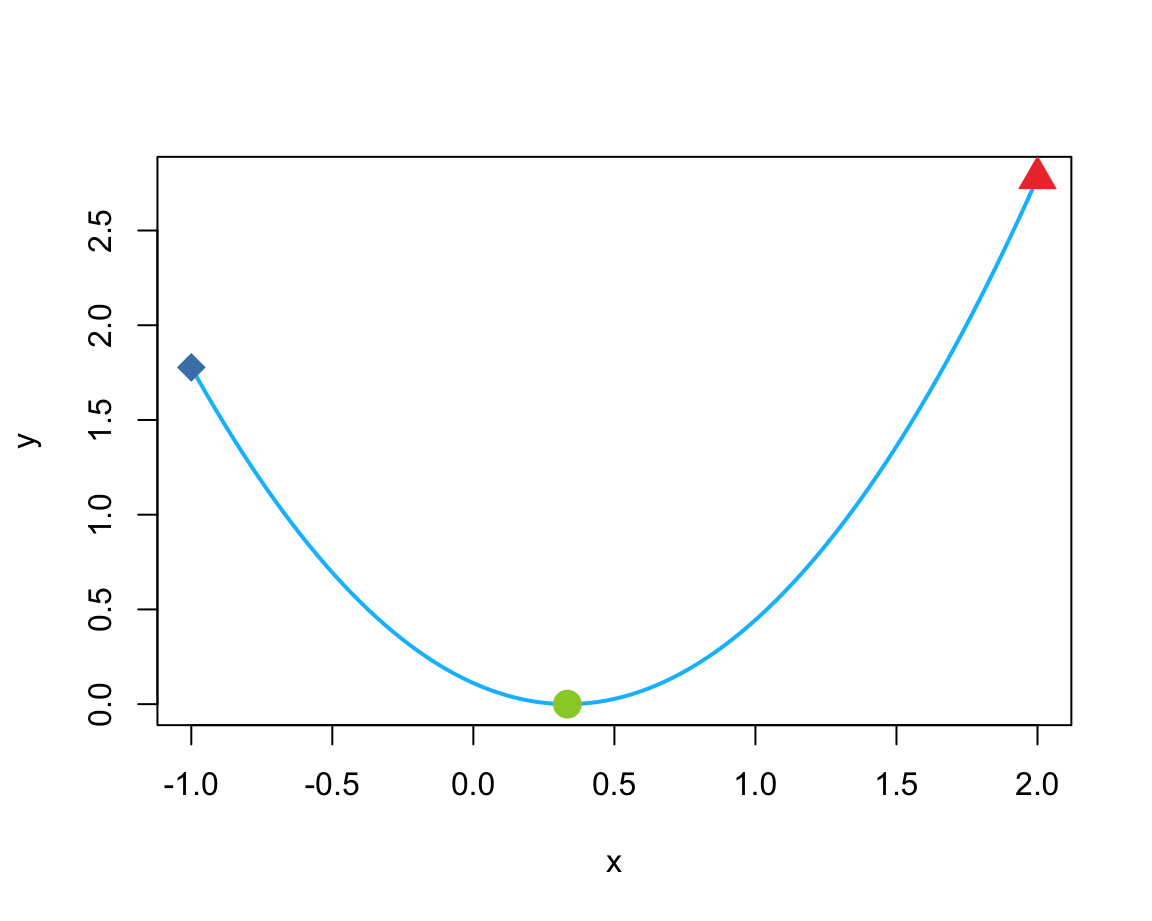Examples based on the documentation of optimize():

# Example 2:
fn <- function(x) {x^3 * (x - 1)}
plot(fn, col = "deepskyblue", lwd = 2)

# Minimum:
(mn <- optimize(fn, lower = 0, upper = 2))
#> $minimum #>  0.7500078 #> #>$objective
#>  -0.1054687
points(x = mn$minimum, y = mn$objective, col = "olivedrab3", pch = 16, cex = 2) # using mx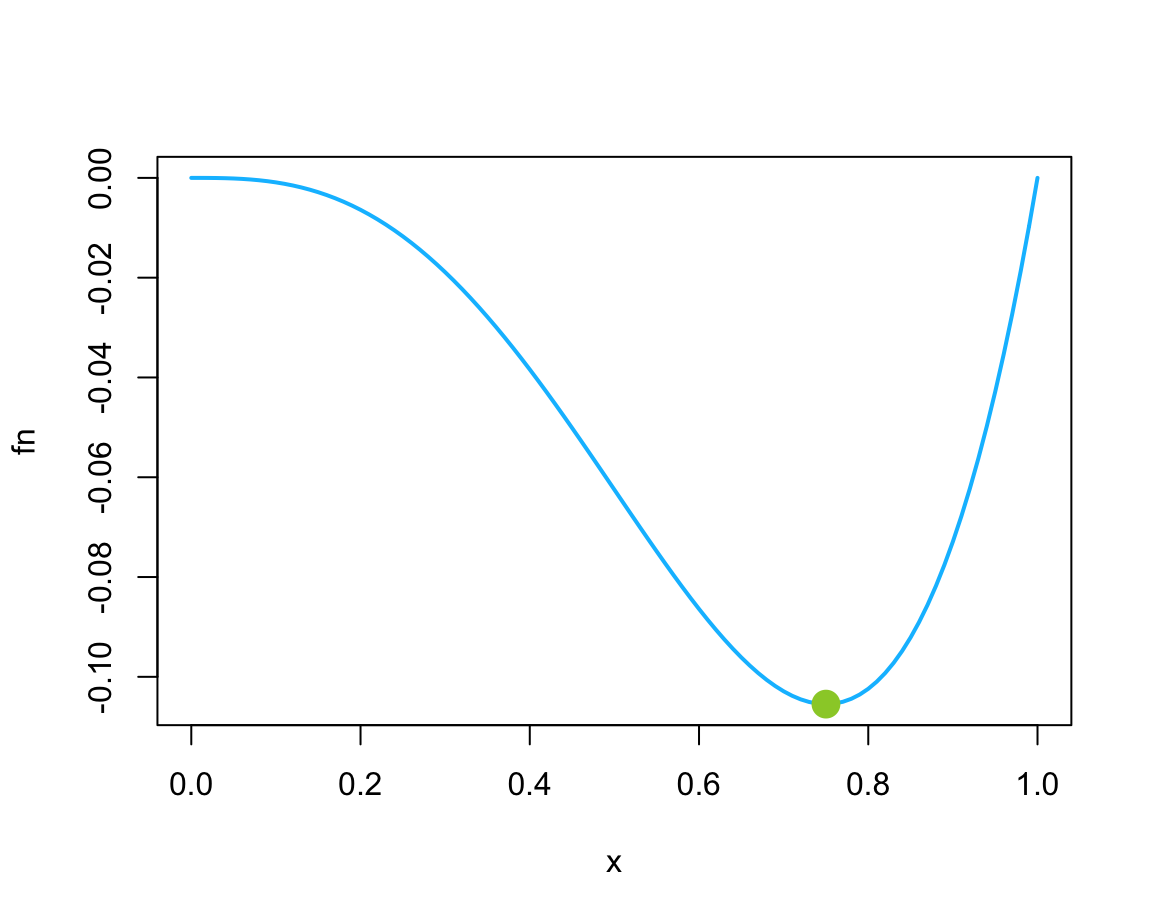# See where a function is evaluated:
optimize(function(x) x^3 * (print(x) - 1), lower = 0, upper = 2)
#>  0.763932
#>  1.236068
#>  0.472136
#>  0.6666667
#>  0.7777988
#>  0.7471242
#>  0.7502268
#>  0.7499671
#>  0.7500078
#>  0.7500485
#>  0.7500078
#> $minimum #>  0.7500078 #> #>$objective
#>  -0.1054687

# Warning:
# A "wrong" solution with an unlucky interval and piecewise constant f():
f  <- function(x) ifelse(x > -1, ifelse(x < 4, exp(-1/abs(x - 1)), 10), 10)
fp <- function(x) { print(x); f(x) }

plot(f, -2,5, ylim = 0:1, col = "red")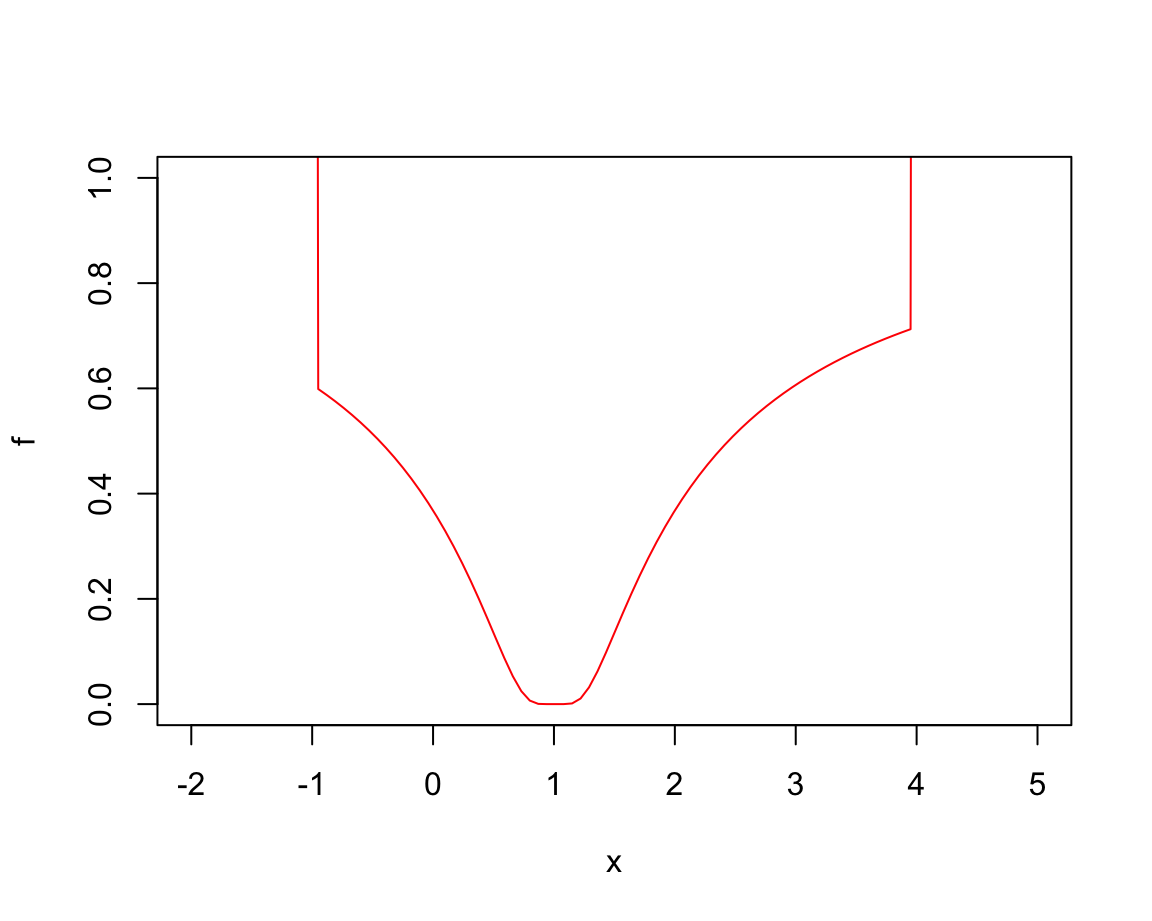optimize(fp, c(-4, 20))   # fails to find the minimum
#>  5.167184
#>  10.83282
#>  14.33437
#>  16.49845
#>  17.83592
#>  18.66253
#>  19.1734
#>  19.48913
#>  19.68427
#>  19.80487
#>  19.8794
#>  19.92547
#>  19.95393
#>  19.97153
#>  19.9824
#>  19.98913
#>  19.99328
#>  19.99585
#>  19.99743
#>  19.99841
#>  19.99902
#>  19.99939
#>  19.99963
#>  19.99977
#>  19.99986
#>  19.99991
#>  19.99995
#>  19.99995
#> $minimum #>  19.99995 #> #>$objective
#>  10
optimize(fp, c(-7, 20))   # finds minimum
#>  3.313082
#>  9.686918
#>  -0.6261646
#>  1.244956
#>  1.250965
#>  0.771827
#>  0.2378417
#>  1.000451
#>  0.9906964
#>  0.9955736
#>  0.9980122
#>  0.9992315
#>  0.9998411
#>  0.9996083
#>  0.9994644
#>  0.9993754
#>  0.9993204
#>  0.9992797
#>  0.9992797
#> $minimum #>  0.9992797 #> #>$objective
#>  0
optimize(fp, c(-2, 5))
#>  0.6737621
#>  2.326238
#>  -0.3475242
#>  0.9935203
#>  1.075221
#>  1.034342
#>  1.013931
#>  1.003726
#>  0.998623
#>  1.001174
#>  0.9998987
#>  1.000538
#>  1.000294
#>  1.000143
#>  1.00005
#>  0.999992
#>  0.9999513
#>  0.9999513
#> $minimum #>  0.9999513 #> #>$objective
#>  0

Here, we explore two different methods (both from the stats package that comes with R): General intuition: Given the scatterplot of our data (shown in Figure 18.1), we have a pretty good idea that we are looking for a linear model.

1. General-purpose optimization (using optim())

2. Fitting a linear model (using lm())

• ad 1: Using optim() to determine the best parameter pair:

Intuition: Given a fn = distance and data, optim() will use an algorithm to find the parameter combination par that minimize the value of distance.

# sim1
best <- optim(par = c(0, 0), fn = distance, data = sim1)
best$par # vector of the 2 best parameters (i.e., minimizing fn for data) #>  4.222248 2.051204 ggplot(sim1, aes(x, y)) + geom_point(color = "steelblue", size = 2, alpha = 2/3) + geom_abline(intercept = best$par, slope = best\$par, color = "gold") +
labs(title = "The best linear model to predict the data",
caption = "Data from modelr::sim1") +
theme_ds4psy()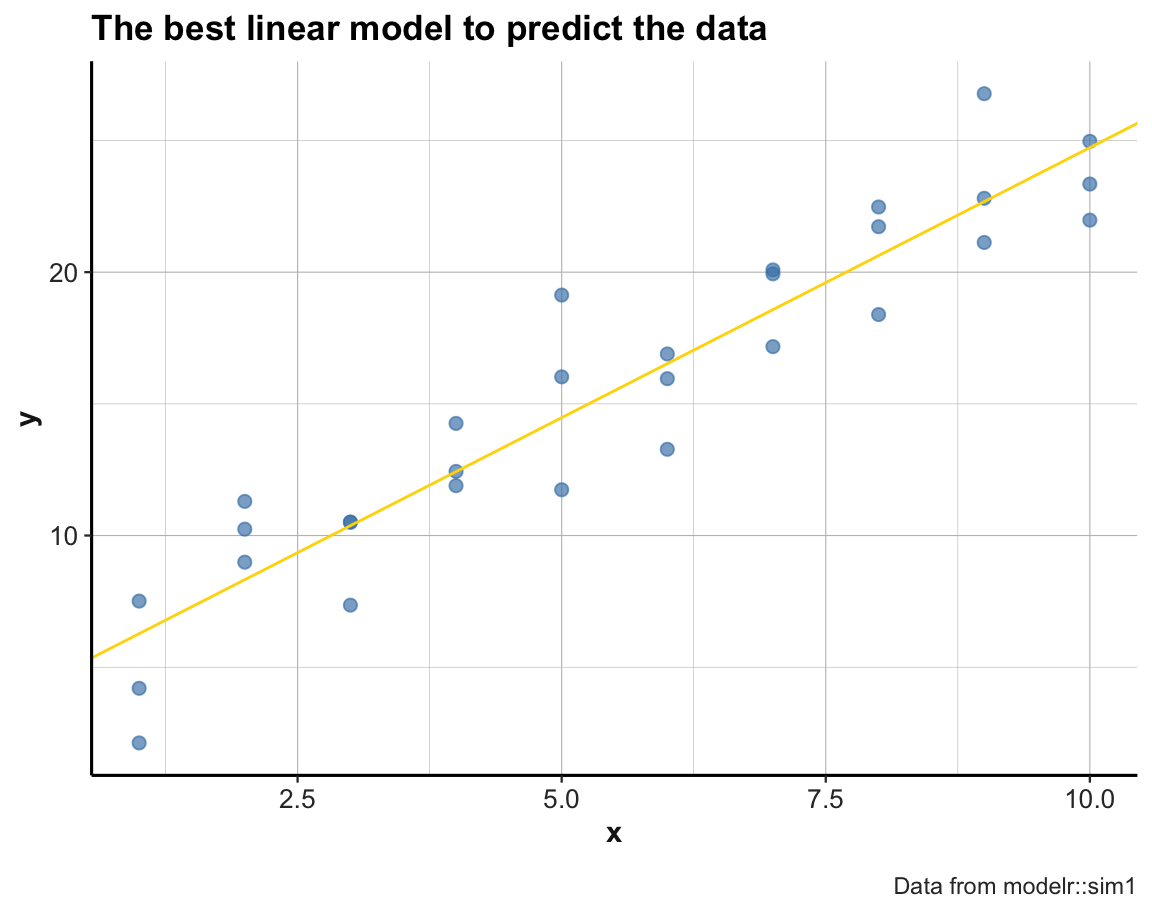• ad 2: Using lm() for fitting a linear model:
sim1_mod <- lm(y ~ x, data = sim1)
coef(sim1_mod)
#> (Intercept)           x
#>    4.220822    2.051533

For fitting other datasets and types of functions, using glm() or nlm() may be indicated.

### 18.3.7 ToDo 2

Quantitative prediction/regression: Generalize and use a more meaningful dataset:

• Candidate data: father.son or galton (both from the UsingR package)

• analysis

See Chapter 3: Linear regression of .

### References

James, G., Witten, D., Hastie, T., & Tibshirani, R. (2021). An introduction to statistical learning with applications in R (2nd edition). Springer. https://www.statlearning.com/
Wickham, H., & Grolemund, G. (2017). R for data science: Import, tidy, transform, visualize, and model data. O’Reilly Media, Inc. http://r4ds.had.co.nz